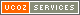Статьи по ДелфиМеню сайтаКатегории каталога
 Мои статьи  Функции и процедуры Win Api  Работа с мышью  Реестр и Делфи  Работа с файлами  Делфи и Хакер  Инсталлятор собственными руками  Хитрости в делфи  Работа с системойФорма входаПоиск по каталогуДрузья сайтаНаш опрос Понравились ли вам треки Первый Да Первый нет Второй Да Второй Нет Понравились оба Оба отстой Всего ответов: 156Приветствую Вас, Гость · RSS 2020-09-19, 10:23 PM

Прочитать и изменить текстовый файл строка за строкой
 procedure TForm1.Button1Click(Sender: TObject); var i, z: Integer; f: TextFile; t: string; Data: array of string; begin if OpenDialog1.Execute then begin //Read line by line in to the array data AssignFile(f, OpenDialog1.FileName); Reset(f); z := 0; SetLength(Data, 0); //Repeat for each line until end of file repeat Inc(z); readln(f, t); SetLength(Data, Length(Data) + Length(t)); Data[z] := t; until EOF(f); SetLength(Data, Length(Data) + 3 * z); //Add to each line the line number for i := 1 to z do Data[i] := IntToStr(i) + ′ ′ + Data[i]; SetLength(Data, Length(Data) + 2); //Add a carriage return and line feed Data := Data + #13 + #10; i := Length(Data); Data := ′′; SetLength(Data, Length(Data) - i); //create a new textfile with the new data AssignFile(f, OpenDialog1.FileName + ′2′); ReWrite(f); //write all lines for i := 1 to z do writeln(f, Data[i]); //save file and close it CloseFile(f); end; end; Категория: Функции и процедуры Win Api | Добавил: Admin (2006-12-16) | Автор: M@ster Просмотров: 1045 | Рейтинг: 0.0 | - Оценить - Отлично Хорошо Неплохо Плохо Ужасно

 Всего комментариев: 0
Имя *:
Email *:
Код *: